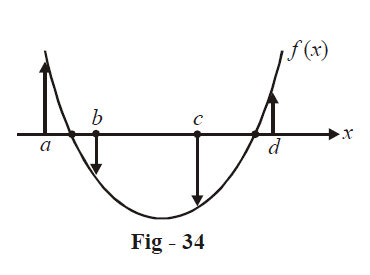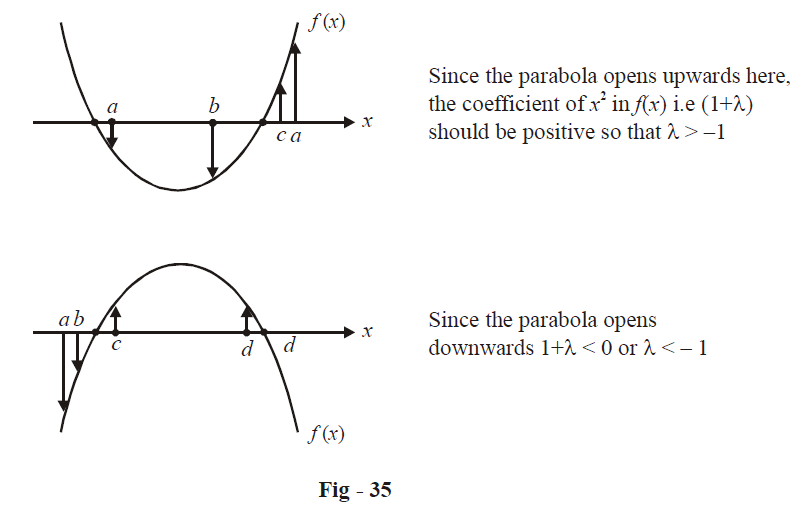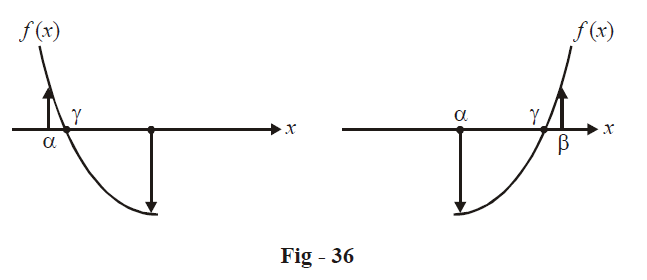Go back to  'SOLVED EXAMPLES'

Example- 1

Solve for x: $$\sqrt {a + \sqrt {a + x} } = x$$

Solution:  Step -1: Square, rearrange, square again:

\begin{align}&a + \sqrt {a + x} = {x^2} \Rightarrow \sqrt {a + x} = {x^2} - a\\&\qquad\qquad\qquad\quad\Rightarrow a + x = {x^4} + {a^2} - 2a{x^2}\end{align}

Step -2: Now, treat this as a (quadratic) equation in a rather than as an equation in x:\begin{align}&\qquad\quad{a^2} - a\left( {1 + 2{x^2}} \right) + {x^4} - x = 0\\ &\Rightarrow\quad a = \frac{{\left( {1 + 2{x^2}} \right) \pm \sqrt {{{\left( {1 + 2{x^2}} \right)}^2} - 4\left( {{x^4} - x} \right)} }}{2}\\\,\,\,\,\,\,\,\,\,\,\,\,\,\,\,\,\,\,\,\, &\qquad\quad= \frac{{\left( {1 + 2{x^2}} \right) \pm \sqrt {4{x^2} + 4x + 1} }}{2}\\\,\,\,\,\,\,\,\,\,\,\,\,\,\,\,\,\,\,\,\, &\qquad\quad= \frac{{\left( {1 + 2{x^2}} \right) \pm \left( {1 + 2x} \right)}}{2}\\\,\,\,\,\,\,\,\,\,\,\,\,\,\,\,\,\,\,\,\, &\qquad\quad= {x^2} - x,\;\;{x^2} + x + 1\end{align}

Step-3: We now have two quadratics:

\begin{align}&{x^2} - x = a\,\,\,\,\,\,\,\,\,\,\,\,\,\,\,\,\,\,\,\,\,\,\,\,\,\,\,\,\,\,\,\,\,\,\,\,\,\,\,{x^2} + x + 1 = a\\ &\Rightarrow x = \frac{{1 \pm \sqrt {1 + 4a} }}{2}\,\,\,\,\, \Rightarrow \,\,\,x = \frac{{ - 1 \pm \sqrt {4a - 3} }}{2}\end{align}

Example- 2

If $${\rm{ }}a\,{\rm{ < }}\,b < c < d$$ then prove that for any real $$\lambda$$ , $$f\left( x \right) = \left( {x - a} \right)\left( {x - c} \right) + \lambda \left( {x - b} \right)\left( {x - d} \right) = 0$$ will always have real roots.

Solution: We can of course follow the approach of writing $$f\left( x \right)$$ in the form of a standard quadratic equation, evaluating its discriminant and proving it to be non-negative for all real values of $$\lambda$$ . However such an approach would become unnecessarily lengthy. Here we will use a graphical approach that is quicker and also gives us more information pertaining to the roots than the discriminant approach.

We consider three cases, $$\lambda = 0,\,\,\lambda > 0\,\,{\rm{and}}\,\,\lambda < 0$$ and show that in all cases, the roots are real. (In graphical terms, this means that in all cases, the graph should intersect the x-axis)

$$\fbox{\(\lambda = 0$$}\)$$\;\;\;\;\;\;\Rightarrow f\left( x \right)=\left( x-a \right)\left( x-c \right)=0$$

This obviously has two real roots,

namely $$x = a,c.$$

$$\begin{array}{l}\fbox{\(\lambda > 0$$} \,\,\,\,\, \Rightarrow \;\;\;\;\;\;\;\;\;\;\;\;\;\;\;\;\;\;& f\left( a \right) = \lambda \left( {a - b} \right)\left( {a - d} \right) > 0\\\,\,&f\left( b \right) = \left( {b - a} \right)\left( {b - c} \right) < 0\\ &f\left( c \right) = \lambda \left( {c - b} \right)\left( {c - d} \right) < 0\\ &f\left( d \right) = \left( {d - a} \right)\left( {d - c} \right) > 0\end{array}\)

Notice that $$f\left( a \right)$$ and$$f\left( b \right)$$ are of opposite sign. This means that the graph has to necessarily cross the x-axis between a and b, or in other words, there is a root of between $$f\left( x \right)$$ a and b. Similarly, there lies another root of $$f\left( x \right)$$ between c and d.

The graph for $$f\left( x \right)$$ is approximately sketched below:We see that the graphical approach also gives us the location of the roots.

\begin{align}&\fbox{$$\lambda = 0$$}\,\,\,\,\,\,\,\,\,\,\,\,\,\,\,\, \Rightarrow \,\,\,\,\,\,f\left( a \right) = \lambda \left( {a - b} \right)\left( {a - d} \right) < 0 \\ \,\,\,\,\,\,\,\,\,\,\,\,\,\,\,\,\,\,\,\,\,\,\,\,\,\,\,\,\,\,\,\,\,\,\,\,\,\,&\qquad\qquad\qquad \quad\; f\left( b \right) = \left( {b - a} \right)\left( {b - c} \right) < 0 \\ \,\,\,\,\,\,\,\,\,\,\,\,\,\,\,\,\,\,\,\,\,\,\,\,\,\,\,\,\,\,\,\,\,\,\,\,\,\,\,&\qquad\qquad\qquad \quad\;f\left( c \right) = \lambda \left( {c - b} \right)\left( {c - d} \right) > 0 \\ \,\,\,\,\,\,\,\,\,\,\,\,\,\,\,\,\,\,\,\,\,\,\,\,\,\,\,\,\,\,\,\,\,\,&\qquad\qquad\qquad \quad\;f\left( d \right) = \left( {d - a} \right)\left( {d - c} \right) > 0 \\\end{align}

Since $$f\left( b \right)\,\,{\rm{and}}\,f\left( c \right)$$ are of the opposite sign, then must be a root of $$f\left( x \right)$$ between b and c. can $$f\left( x \right)$$ have the following configurations.Here also, since the graph of $$f\left( x \right)$$ intersects the x-axis, $$f\left( x \right)$$ has real roots. In addition, notice that the graph also tells us that if $$\lambda > - 1,$$ the second root lies to the left of a while if $$\lambda < - 1,$$ the second root lies to the right of d. (What if $$\lambda = - 1$$ ?)

In all cases, $$f\left( x \right)$$ has real roots

Example- 3

If $$\alpha$$ is a real root of the quadratic equation $$a{x^2} + bx + c = 0$$ and $$\beta$$ is a real root of $$- a{x^2} + bx + c = 0,$$ show that there is a root $$\gamma$$ of the equation \begin{align}\frac{a}{2}{x^2} + bx + c = 0\end{align} which lies between $$\alpha$$ and $$\beta$$ .

Solution: Let us denote $$\frac{a}{2}{x^2} + bx + c$$ by $$f\left( x \right).$$

Since we want \begin{align}\gamma \,\left( {{\rm{a}}\,{\rm{root}}\,{\rm{of}}\,f\left( x \right)} \right)\end{align} to lie between $$\alpha$$ and $$\beta$$, our approach should be to somehow show that $$f\left( \alpha \right)\,{\rm{and}}\,f\left( \beta \right)$$ are of opposite sign, so that the graph of $$f\left( x \right)$$ crosses the x-axis between $$\alpha \,{\rm{and}}\,\beta .$$

The figure below illustrates this point:Now, since $$\alpha$$ is a root of $$a{x^2} + bx + c = 0$$

\begin{align}& \Rightarrow a{\alpha ^2} + b\alpha + c = 0\\ &\Rightarrow \frac{a}{2}{\alpha ^2} + b\alpha + c = - \frac{a}{2}{\alpha ^2}\\ &\Rightarrow f\left( \alpha \right) = - \frac{a}{2}{\alpha ^2} \;\;\;\;\;\;\ldots (i)\end{align}

Since $$\beta$$ is a root of $$- a{x^2} + bx + c = 0$$

\begin{align}&\Rightarrow - a{\beta ^2} + b\beta + c = 0\\ &\Rightarrow \frac{a}{2}{\beta ^2} + b\beta + c = \frac{{3a}}{2}{\beta ^2}\\ &\Rightarrow f\left( \beta \right) = \frac{{3a}}{2}{\beta ^2} \;\;\;\;\;\;\;\;\ldots (ii)\end{align}

From (i) and (ii) notice that $$f\left( \alpha \right)$$ and $$f\left( \beta \right)$$ are of opposite signs and hence a root $$\gamma$$ of $$f\left( x \right)$$ lies between $$\alpha$$ and $$\beta$$

Example- 4

Find the range of values that \begin{align}f\left( x \right) = \frac{{{x^2} - 3x + 4}}{{{x^2} + 3x + 4}}\end{align} can assume, for real values of x.

Solution: This question is similar to examples 6 and 7 of the unit on Functions (Page - 19). We put $$f\left( x \right) = y,$$ find x in terms of y and find those values of y for which x is real. These possible values of y form the range of $$f\left( x \right)$$ that we wish to determine.

\begin{align}&\qquad\quad f\left( x \right) = \frac{{{x^2} - 3x + 4}}{{{x^2} + 3x + 4}} = y\\ &\Rightarrow\quad \left( {1 - y} \right){x^2} - 3\left( {1 + y} \right)x + 4\left( {1 - y} \right) = 0 \;\;\;\;\;\ldots (i)\end{align}

Since we want x to be real, the D of the (i) above must be non-negative (this condition places a restriction on the values that y can take and hence gives us the range)

\begin{align}& D \ge 0\\\\ &\Rightarrow {\left( {3\left( {1 + y} \right)} \right)^2} \ge 16{\left( {1 - y} \right)^2}\\\\ &\Rightarrow 9{y^2} + 18y + 9 \ge 16{y^2} + 16 - 32y\\\\ &\Rightarrow 7{y^2} - 50y + 7 \le 0\\\\ &\Rightarrow \left( {7y - 1} \right)\left( {y - 7} \right) \le 0\\\\ &\Rightarrow \frac{1}{7} \le y \le 7\end{align}

For these values of y, x is real in (i). Hence $$f\left( x \right)$$ can take only these values. Our range is

$R = \left[ {\frac{1}{7},7} \right]$

Learn from the best math teachers and top your exams

• Live one on one classroom and doubt clearing
• Practice worksheets in and after class for conceptual clarity
• Personalized curriculum to keep up with school